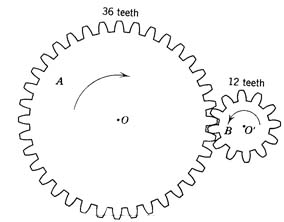#COURSE DESCRIPTION: The main focus of this class is on solving problems involving linear, quadratic and exponential relations with an introduction to the concepts of functions and mathematical modeling.  Topics include solving and graphing linear, quadratic and exponential equations, systems of linear equations, rational exponents, root equations, quadratic inequalities, the distance and midpoint formulas, circles exponential growth/decay.

The "Rule of Three" applies: any problem in mathematics can be addressed through the analysis of

(1) tabulated data
(2) visual displays (graphs)
(3) equations involving operations on numbers and variables.

Topics include

§  systems of linear and quadratic equations in several variables

§  functions

§  powers, root and polynomials functions

§  exponential/logarithmic functions

§  circles, parabolas and other conic sections

TEXTBOOK:
Intermediate Algebra, Functions, and Graphs by Yoshiwara.
This text places emphasis on the use of basic algebra in mathematical modeling and problem solving. Topics include using linear, quadratic, root, exponential and logarithmic functions and elementary conic sections for graphing and modeling. The assigned reading and problems are crucial and it will be obvious that you haven’t done it if you are not prepared with questions about these in class. We will not be able to cover every problem type or every approach during class, but you will be responsible for everything discussed in the text, so if there is something you don’t get, it’s up to you to be aware of that and to ask questions to resolve any confusion you can articulate.

LECTURE/HOMEWORK/ LABS :
Generally, once you've read the exposition and basic definitions and you can expect the most important part of your learning to come from applying these to the problems assigned for homework and lab time.  Of course, you will have a much easier time doing homework and performing well on exams if you pay attention and take excellent notes during lecture. Complete the homework assignment for each section to be well prepared for quizzes and exams. Do assignments to the best of your ability before the next class and have specific questions about places where you become stuck or confused.  You should be prepared at the beginning of each class with questions from the homework.

Note: You will be responsible for keeping a homework notebook that can be presented on demand, but you will also need to log onto the Cengagenow homework system at http://www.ilrn.com/ilrn/ and complete regular assignments there.

EXAMS:
There are 7 chapter tests scheduled (one after each chapter) and a cumulative final exam. Keep in mind that the point of these exams is to test your understanding of the concepts covered; that is, they will not necessarily consist of just homework problems with the numbers changed, but may involve novel problems testing your understanding of how to use basic principles of algebra in context.

CALCULATORS:  A scientific calculator is required for this course. Though the text book devotes a large amount of material to using a graphing calculator, a graphing calculator is not required for this class.  Whenever textbook problems request the use a graphing calculator (as is often the case), pencil and paper is all you will need.

ATTENDANCE:  You are required to attend all lecture and lab sessions: 6 hours per week for 16 weeks: a total of 96 hours.  The two most significant indicators for success in this course are attendance and completion of the homework. If you're not there doing what it is that defines the class, you missed it.

GRADE:  Your grade in this class will be a weighted average of your attendance, homework quiz, chapter test and final exam scores, using the following apportionment:

Attendance/quiz: 10 pts.
Homework: 10 pts.
Chapter tests: 50 pts.
Final Exam: 30 pts.

Course Objectives:

Upon successful completion of this course you will be able to

• Comprehend that the key characteristic of a linear model is its constant rate of change.
• Interpret slope as a rate of change.
• Recognize when a table, graph, or equation is linear.
• Create a linear model in the form of a table, graph, or equation.
• Find the equation of a line and apply it to solve problems with a constant of change.
• Solve 2x2 and 3x3 systems of linear equations.
• Graph systems of linear inequalities in two dimensions.
• Graph and find the equation of a circle.
• Solve quadratic equations by factoring, completing the square, taking square roots or the quadratic formula.